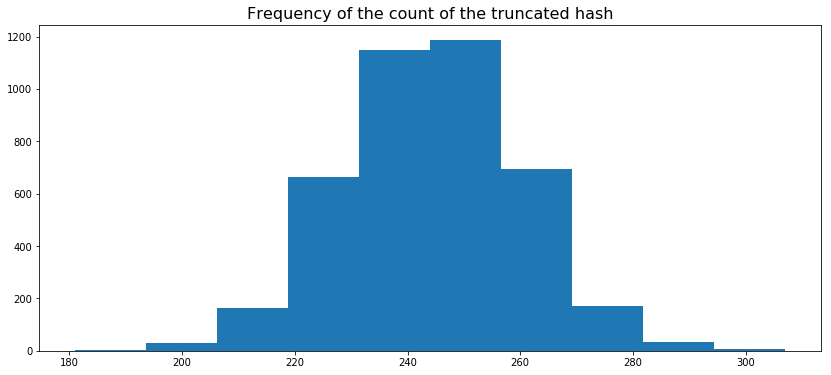Fisseha Berhane, PhD

Sampling using truncated hash¶

Let's suppose tens of millions of people visit your website everyday and you want to do adhoc analysis. However, you cannot use all the data since it is computationally costly and also jobs will take long time. Therefore, a better approach is to have a representative sample. But how can you sample from the data so that a visitor that has been included in today's sample is also included in tomorrow's sample or anytime in the future? In this short post, we will see how to attain that using truncated hash.

But how is truncated hash applicable in this case?¶

When we apply a hash to the IDs of the website visitors and truncate the first few characters of the hash, the decimal value of the truncated hash is uniformly distributed. Therefore, we can sample from the truncated hash and use those samples to get sample data anytime in the future. Note here that we have to change the truncated hash from hexadecimal to decimal (base 10) to generate the density and cumulative distribution plots. In the code below, we will use the first three characters of the hash of the users. In doing so, we get 4096 (16*16*16) unique truncated hash values which can be used as buckets for our sampling.

Create Spark session, import necessary packages and functions¶

In :
import findspark
findspark.init()

import uuid
import numpy as np
import matplotlib.pyplot as plt
from statsmodels.distributions.empirical_distribution import ECDF
import pandas as pd
from pyspark.sql.functions import *
from pyspark.sql.types import *
%matplotlib inline

from pyspark.sql import SparkSession
spark = SparkSession.builder.getOrCreate()

Generate 1 million universaly unique identifiers (UUIDs)¶

In the code below, I created md5 hash from the UUIDs and then truncated the first three characters of each hash. Finally, I converted the first three characters from hex to decimal (from base 16 to base 10) using the conv function. As you can see, I have converted them to integer using cast(IntegerType()).

In :
uuid1 = [str(uuid.uuid1()) for i in range(1000000)]
uuid1_df = pd.DataFrame({'uuid1':uuid1})

uuid1_spark_df =  spark.createDataFrame(uuid1_df)

uuid1_spark_df = uuid1_spark_df.withColumn('hash', md5(col('uuid1')))\
.withColumn('truncated_hash3', substring(col('hash'), 1, 3))\
.withColumn('decimal_value', conv('truncated_hash3', 16, 10).cast(IntegerType()))

uuid1_spark_df.show(10)   # show sample data
+--------------------+--------------------+---------------+-------------+
|               uuid1|                hash|truncated_hash3|decimal_value|
+--------------------+--------------------+---------------+-------------+
|6bdae40c-d7fc-11e...|a78a18e19645a32c0...|            a78|         2680|
|6bdae646-d7fc-11e...|0c6342c0b76314920...|            0c6|          198|
|6bdae696-d7fc-11e...|1e444d60dc27e7553...|            1e4|          484|
|6bdae6e6-d7fc-11e...|db2d6f9e99585ea06...|            db2|         3506|
|6bdae70e-d7fc-11e...|3f6cf6936f7cea199...|            3f6|         1014|
|6bdae72c-d7fc-11e...|941539ffdbba953c3...|            941|         2369|
|6bdae754-d7fc-11e...|70e2f9981c06cef94...|            70e|         1806|
|6bdae772-d7fc-11e...|80593f29bec536c8d...|            805|         2053|
|6bdae790-d7fc-11e...|1dfb05a431b531e69...|            1df|          479|
+--------------------+--------------------+---------------+-------------+
only showing top 10 rows

Plot the distribution¶

Now, let's plot the distribution of the decimal value of the first three characters. Are they uniformly distributed?

In :
decimal_values_array  = uuid1_spark_df.select('decimal_value').toPandas().values

plt.figure(figsize = (14, 6))
plt.hist(decimal_values_array, alpha = 0.5)
plt.title('Histogram of truncated hash converted to decimal', size = 16)
plt.show()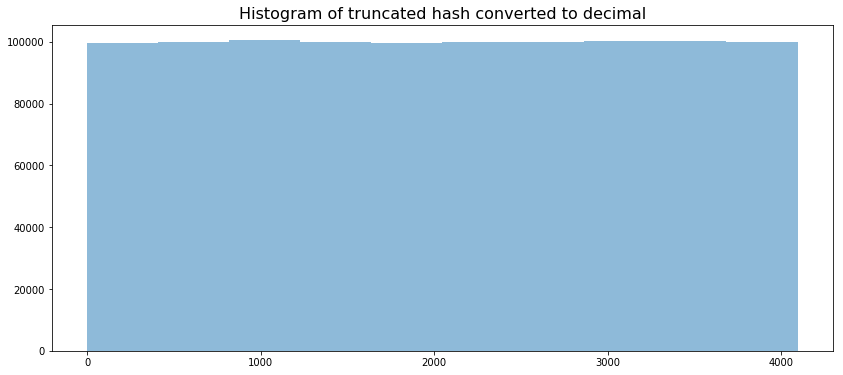As you can see from the histogram above, our data is uniformly distributed.

Let's generate random numbers from a uniform distribution and see if the histograms are similar.

In :
generated_data = np.random.uniform(low = 0, high = 4096, size = 1000000)
plt.figure(figsize = (14, 6))
plt.hist(generated_data, alpha = 0.5)
plt.title('Histogram of data generated from a uniform distribution', size = 16)
plt.show()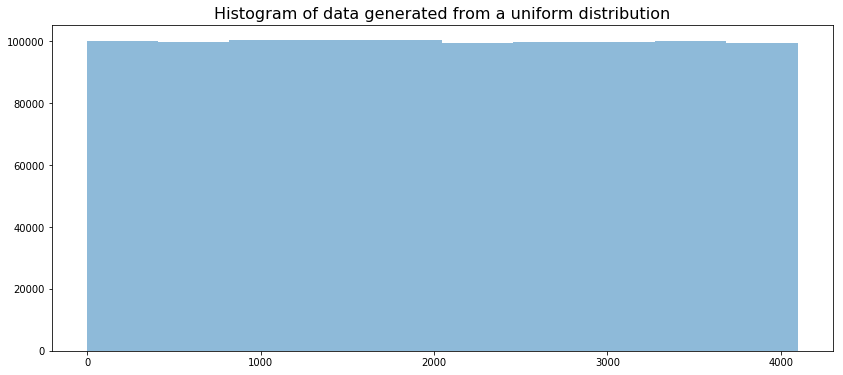The histograms are very similar.

We can also check the cumulative distribution and compare it with the cumulative distibution of the data generated from a uniform distribution.

Cumulative Distribution¶

In :
ecdf = ECDF([item for item in uuid1_spark_df.select('decimal_value').collect()])
plt.figure(figsize = (14, 6))
plt.plot(ecdf.x,ecdf.y)
plt.title('Cumulative distribution of truncated hash converted to decimal', size = 16)
plt.show()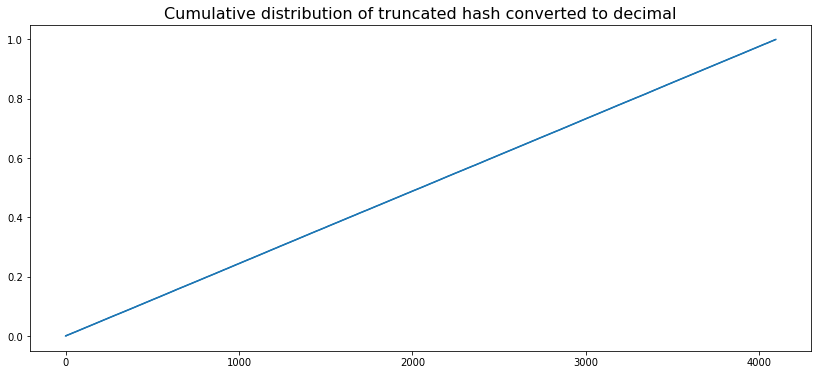And below is the cumulative distribution of the data generated from a uniform distribution. As you can see, it is similar to the cumulative distribution above.

In :
ecdf = ECDF(generated_data)
plt.figure(figsize = (14, 6))
plt.plot(ecdf.x,ecdf.y)
plt.title('Cumulative distribution of data generated from a uniform distribution', size = 16)
plt.show()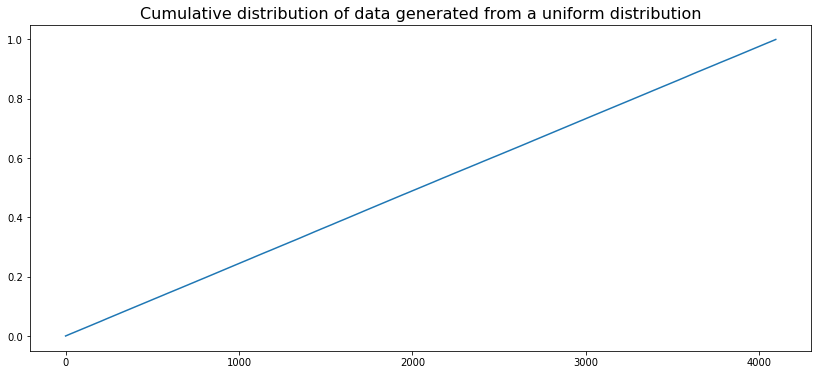We can also see the bar graph ot the truncated_hash3 (which is the truncated hash in hexadecimal). We expect each one of them to have similar frequencies.

In :
truncated_hash3_count  = uuid1_spark_df.groupBy('truncated_hash3').count().toPandas()
Out:
truncated_hash3 count
0 99a 239
1 675 242
2 296 215
3 7ce 247
4 edd 240
In :
truncated_hash3_count.plot(figsize = (20, 10),
y = 'count', x = 'truncated_hash3', kind  = 'bar', legend = False)
plt.xticks(fontsize =0)
plt.xlabel('Truncated Hash (Hex)', size = 14)
plt.yticks(size = 14)
plt.title("Frequency of each truncated hash", fontsize = 16)
plt.show()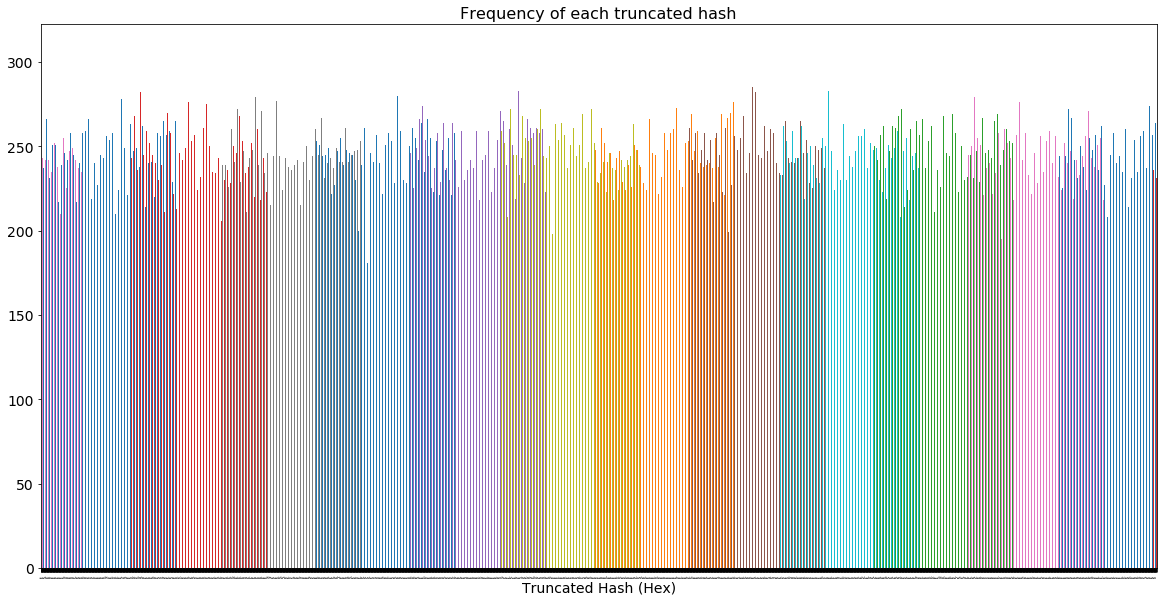By the way, the count of each truncated hash shown in the bar graph above is nearly normally distributed. From the bar graph above, we see most of them are concentrated around 244 but there are some truncated hash values which have frequency above and below 244 and hence we get the nomal distribution of their counts shown below.

In :
plt.figure(figsize = (14, 6))
plt.hist(truncated_hash3_count['count'].values)
plt.title("Frequency of the count of the truncated hash", fontsize = 16)
plt.show()